Courses

# RD Sharma Solutions Ex-6.1, Factorization Of Polynomials, Class 9, Maths Class 9 Notes | EduRev

## Class 9 : RD Sharma Solutions Ex-6.1, Factorization Of Polynomials, Class 9, Maths Class 9 Notes | EduRev

The document RD Sharma Solutions Ex-6.1, Factorization Of Polynomials, Class 9, Maths Class 9 Notes | EduRev is a part of the Class 9 Course RD Sharma Solutions for Class 9 Mathematics.
All you need of Class 9 at this link: Class 9

Q1. Which of the following expressions are polynomials in one variable and which are not?

1. 3x2–4x+15
2. y2+2√3
3. 3√x+√2x
4.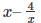5. x12+y2+t50

Sol :

1. 3x2–4x+15 – it is a polynomial of x

2. y2+2√3 – it is a polynomial of y

3. 3√x+√2x – it is not a polynomial since the exponent of 3√x is not a positive term

4.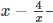it is not a polynomial since the exponent of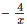is not a positive term

5. x12+y2+t50 – it is a three variable polynomial which variables of x, y, t

Q2. Write the coefficients of x2 in each of the following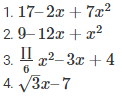Sol :

Given , to find the coefficients of x2

1. 17–2x+7x2 – the coefficient is 7

2. 9–12x+x2 – the coefficient is 0

3.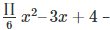the coefficient is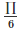4.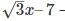the coefficient is 0

Q3. Write the degrees of each of the following polynomials :

1. 7x3+4x2–3x+12
2. 12–x+2x2
3. 5y–√2
4. 7−7x0
5. 0

Sol :

Given , to find degrees of the polynomials

Degree is highest power in the polynomial

1. 7x3+4x2–3x+12 – the degree is 3

2. 12–x+2x3 – the degree is 3

3. 5y–√2 – the degree is 1

4. 7−7x0 – the degree is 0

5. 0 – the degree of 0 is not defined

Q4. Classify the following polynomials as linear, quadratic, cuboc and biquadratic polynomials :

1. x+x2+4
2. 3x – 2
3. 2x+x2
4. 3y
5. t2+1

f . 7t4+4t2+3t–2

Sol :

Given

1. x+x2+4 – it is a quadratic polynomial as its degree is 2

2. 3x – 2 – it is a linear polynomial as its degree is 1

3. 2x+x2 – it is a quadratic polynomial as its degree is 2

4. 3y – it is a linear polynomial as its degree is 1

5. t2+1 – it is a quadratic polynomial as its degree is 2

f . 7t4+4t2+3t–2 – it is a bi- quadratic polynomial as its degree is 4

Q5. Classify the following polynomials as polynomials in one variables, two – variables etc :

1. x2–xy+7y2
2. x2–2tx+7t2–x+t
3. t3–3t2+4t–5
4. xy + yz + zx

Sol :

Given

1. x2–xy+7y2 – it is a polynomial in two variables x and y

2. x2–2tx+7t2–x+t – it is a polynomial in two variables x and t

3. t3–3t2+4t–5– it is a polynomial in one variable t

4. xy+yz+zx – it is a polynomial in 3 variables in x , y and z

Q6. Identify the polynomials in the following :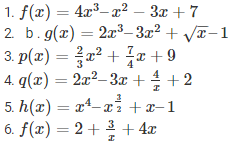Sol :

Given

1. f(x) = 4x3–x2−3x+7 – it is a polynomial

2. b. g(x) = 2x3–3x2+√x–1 – it is not a polynomial since the exponent of √x is a negative integer

3.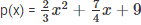it is a polynomial as it has positive integers as exponents

4.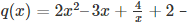it is not a polynomial since the exponent of 4/x is a negative integer

5.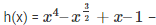it is not a polynomial since the exponent of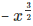is a negative integer

6.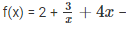it is not a polynomial since the exponent of 3/x is a negative integer

Q7. Identify constant , linear , quadratic abd cubic polynomial from the following polynomials :

1. f(x) = 0
2. g(x) = 2x3–7x+4
3.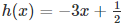4. p(x) = 2x2–x+4
5. q(x) = 4x+3
6. r(x) = 3x3+4x2+5x–7

Sol :

Given ,

1. f(x) = 0 – as 0 is constant , it is a constant variable

2. g(x) = 2x3–7x+4 – since the degree is 3 , it is a cubic polynomial

3.– since the degree is 1 , it is a linear polynomial

4. p(x) = 2x2–x+4 – since the degree is 2 , it is a quadratic polynomial

5. q(x) = 4x+3 – since the degree is 1 , it is a linear polynomial

6. r(x) = 3x3+4x2+5x–7 – since the degree is 3 , it is a cubic polynomial

Q8. Give one example each of a binomial of degree 25, and of a monomial of degree 100

Sol :

Given , to write the examples for binomial and monomial with the given degrees

Example of a binomial with degree 25 – 7x35–5

Example of a monomial with degree 100 – 2t100

Offer running on EduRev: Apply code STAYHOME200 to get INR 200 off on our premium plan EduRev Infinity!

91 docs

,

,

,

,

,

,

,

,

,

,

,

,

,

,

,

,

,

,

,

,

,

,

,

,

,

,

,

,

,

,

;